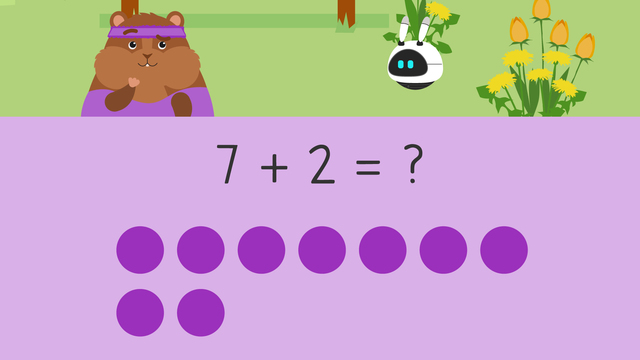# More and Fewer (to ten)

Content More and Fewer (to ten)Rating

Ø 3.0 / 9 ratings
The authorsTeam Digital
More and Fewer (to ten)
CCSS.MATH.CONTENT.1.OA.C.5

## What Does More Than Mean in Math?

What does the word more and fewer mean? Let’s look at the definition of the term more in math:

When someone uses the word more, they are adding to what you already have. We use the word more in math to talk about addition.

What does the word fewer mean?

When someone uses the word fewer, they are saying you have less or you are taking away from something. When you add or subtract, you can count on or back to help.

## More or Fewer in Math Problems

Using the words more and fewer can help to understand what is needed to do to solve a problem. When solving addition and subtraction equations, you can determine whether the result is more or less by following these steps:

Step # What to do
1 Start by creating an equation
2 Count on if the equation
3 Count back if the equation
uses less or subtraction.
4 Finally, solve to find the answer!

## More or Fewer – Example

Imagine you had a box with eighteen screws, but you actually gave five away, so you now have fewer screws. Does fewer mean less or more? Fewer means less, so we need to subtract here. First set up an equation. Here the equation is eighteen minus five equals what? Next, count on or back. We need to count back because this is a subtraction problem.What is eighteen minus five? Thirteen! Let’s practice again!

Imagine you have a box with twenty bolts and someone gives you seven more. When we use the word more, we are talking about an addition equation. First set up an equation, here it is twenty plus seven equals what? Next, count on or back. We need to count on because this is an addition problem.What is twenty plus seven? Twenty-seven!

## More or Fewer – Summary

Today we learned about the terms more and fewer. We learned that the word more is used to add something to what you already have, and is usually used in addition equations. We also learned that the word fewer is used to take away from what you already have, and is usually used in subtraction equations. We also added and subtracted by creating equations and counting on or counting back afterwards to solve the equation. Here are the steps recapped for repetition. When you are asked if something is more or fewer, you should keep these steps in mind:

• Create an equation

• Count on or count back

• Solve the equation to find the answer.

Looking for extra practice with more and fewer? Check out the interactive exercises, worksheets and other activities on more and fewer.

## More and Fewer (to ten) exercise

Would you like to apply the knowledge you’ve learned? You can review and practice it with the tasks for the video More and Fewer (to ten).
• ### Match the story problem to the equation.

Hints

The dots help show the problem. You can count the dots to help figure out the equation.

What does more tell you? Is this a subtraction or addition problem?

Solution

4 + 3

• More tells you to use addition.
• There are 4 dots on the left and 3 dots on the right, so the equation is 4 + 3.
• There are 7 dots in total and 4 + 3 = 7
• ### Arrange the steps to solving a problem.

Hints

Before you count on or back, what is the first step?

What is the last thing you need to do after you have found the solution?

Solution
1. Write the equation.
2. Starting with the larger number, count on or back the smaller number.
3. Find the solution.
4. Write the solution after the equal sign.
• ### Match the equation to the story problem.

Hints

What does fewer tell you to do? Should you add or subtract?

Start at the larger number, 5, and count back by 2. How many dots are shaded dark purple?

Solution

5 - 2 = 3

• Fewer means to subtract.
• Start at 5 and count back 2.
• The total is 3.
• ### Complete the addition number sentence.

Hints

Count the light purple and dark purple dots to help you find the equation.

Start at the larger number to count on to find the solution.

There are two amounts to add here, to find the total.

Solution

6 + 3 = 9 3 + 6 = 9

• Start at 6 and count on 3.
• The total is 9.
• ### Determine which type of equation it is.

Hints

Use the operation symbol to determine whether you need to add or subtract.

Solution

All of the equations with the plus sign are addition problems.

• 5 + 4 = 9
• 3 + 3 = 6
• 5 + 5 = 10
All of the equations with the minus sign are subtraction problems.

• 10 - 1 = 9
• 7 - 3 = 4
• 6 - 4 = 2
• ### Find the matching equation pairs.

Hints

Count on or back depending on which type of equation it is.

If the equation has a + symbol, count on.

If the equation has a - symbol, count back.

Solution
• 6 + 4 = 10 Start at 6 and count on 4. The total is 10.
• 9 + 1 = 10 Start at 9 and count on 1.The total is 10.
• 7 - 5 = 2 Start at 7 and count back 5.The total is 2.
• 8 - 1 = 7 Start at 8 and count back 1. The total is 7.